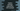# Python Package : Python Tutorial 19## Python Package :

What is a python package ? In simple words , python packages are directories containing python files. We love to arrange files in our computer in different directories, like all movies in a “movie” folder or all songs in a “songs” folder etc.

So, what is the use of using packages ? We can keep similar files in the same folder. If your application is of large number of files, then don’t you think that it would be better to place related files in a same folder instead of placing in separate folders ?

Inside a folder or package , we can create another package ,known as sub-package. Similarly , inside a sub-package , we can also have another package.

## How Does python recognises package :

As packages are simply folders, how does python know these folders are python - packages ? Let’s take a look how to create a python package :

1. Create a folder and place your python files (.py files ) inside it .
2. Create one** init.py** file inside the folder.

i.e. if a folder contains init.py” file, it is a package.

## Example of python package :

Let’s create a directory “projectdir”. Inside this directory, create one file “main.py”. Now create two more directories “packageone” and “packagetwo”. Inside “packageone” create two files : “_init.py_” and “one.py” . And inside “packagetwo” create one file : “**two.py_**”. So the structure will be like :

``````/project_dir :
—— main.py
—— /package_one
— __init__.py
— one.py
—— /package_two
— two.py``````

So, “packageone_ “ directory is a package . Now we will try to call “one.py” from “main.py” and “one.py” from “two.py” .

Edit one.py as :

``````def func_one():
print "inside function one"``````

Now to call “funcone” of “one.py” from “main.py_”, we need to import it first :

`from package_one.one import func_one` and after that we can call “funcone_” .

Edit “main.py” as below :

``````from package_one.one import func_one
func_one()``````

It will print `Inside function one` .  So, you have seen that we can directly call a function of a package by importing the package . But , how can we call “one.py” from “two.py” ? Both are in the same level !!

using “sys.path.append” . Edit “two.py” as below :

``````import sys
sys.path.append('../')
from package_one.one import func_one

func_one()``````

Now , if you run “two.py” , it will provide the output  `“Inside function one “`.

Actually, python check for packages in all directories defined in “sys.path”. So, we have appended the parent path to “sys.path” .